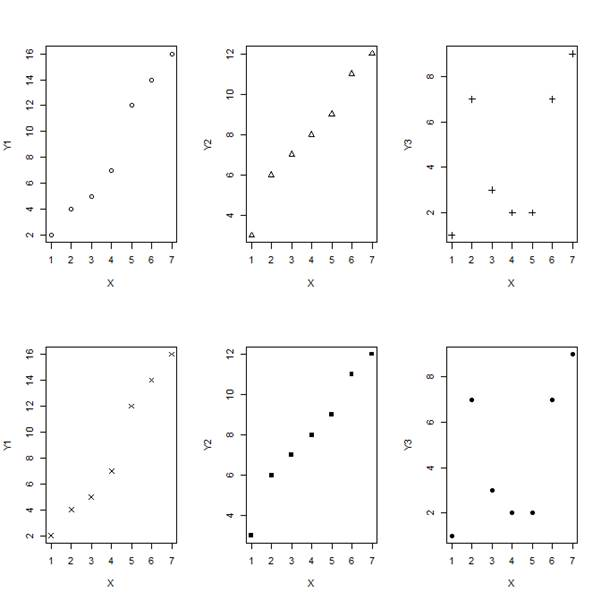# R Graphics: Multiple Graphs and par(mfrow=(A,B))Today we see how to set up multiple graphs on the same page. We use the syntax ` par(mfrow=(A,B))`

. . . where A refers to the number of rows and B to the number of columns (and where each cell will hold a single graph). This syntax sets up a plotting environment of A rows and B columns.

First we create four vectors, all of the same length.

`X <- c(1, 2, 3, 4, 5, 6, 7)`

`Y1 <- c(2, 4, 5, 7, 12, 14, 16)`

`Y2 <- c(3, 6, 7, 8, 9, 11, 12)`

`Y3 <- c(1, 7, 3, 2, 2, 7, 9)`

Now we set up a plotting environment of two rows and three columns (in order to hold six graphs), using `par(mfrow())`

`par(mfrow=c(2,3)) `

Now we plot six graphs on the same plotting environment. We use the `plot()` command six times in succession, each time graphing one of the Y vectors against the X vector.

`plot(X,Y1, pch = 1)`

`plot(X,Y2, pch = 2)`

`plot(X,Y3, pch = 3)`

`plot(X,Y1, pch = 4)`

`plot(X,Y2, pch = 15)`

`plot(X,Y3, pch = 16)`

Out plot looks like this:That wasn’t so hard! In our next blog post we will learn about some useful commands for exploring data in R.

About the Author: David Lillis has taught R to many researchers and statisticians. His company, Sigma Statistics and Research Limited, provides both on-line instruction and face-to-face workshops on R, and coding services in R. David holds a doctorate in applied statistics.Getting Started with R
Kim discusses the use of R statistical software for data manipulation, calculation, and graphical display.

1.Shailaja Chadha says

Hello,
Is there a way to do this for a very large data set so that you don’t have to type in
plot(X,Y3, pch = 16)…. and so on.

I want a way to generate scatter plots for all columns (y=column heading) against the treatments (x=treatments).

Thanks so much.

2.Mohammad says

Thanks.
Is there any way we can change the margin of the plots? i mean They all have same border and margins atm, I want one of my plots to have less space.

3.Kul says

Is there a way to write a name for all plots? E.g. in your example how could one write “Two rows of Y plots” on top of the plot/file?

•Mohammad says

e.g.:
plot(X,Y, main=”title”)

4.Sam Jake says

Thank you. Is there a way, where multiple graphs can be plotted in the same x & y axis?

•Cindy says

You want

par(new=F)

This will not erase the existing plot before drawing the new one. Note however that it does not attempt to scale the overlaid plot to the existing one – you can either use different y-axes on either side of the plot, or scale the overlaid plot yourself. Otherwise your new plot will be the right shape, but appear to have the wrong y-values.

Please note that, due to the large number of comments submitted, any questions on problems related to a personal study/project will not be answered. We suggest joining Statistically Speaking, where you have access to a private forum and more resources 24/7.